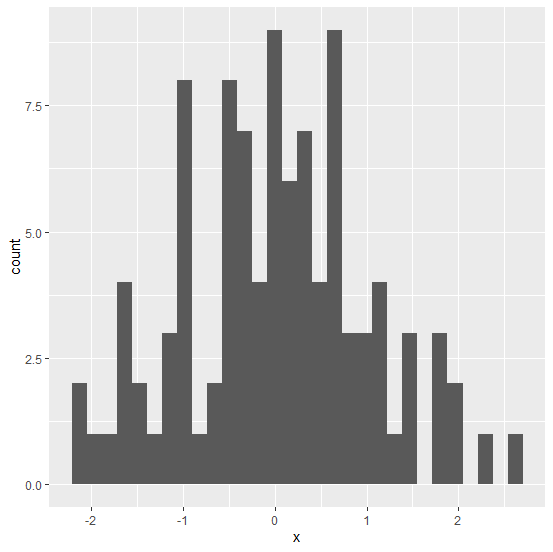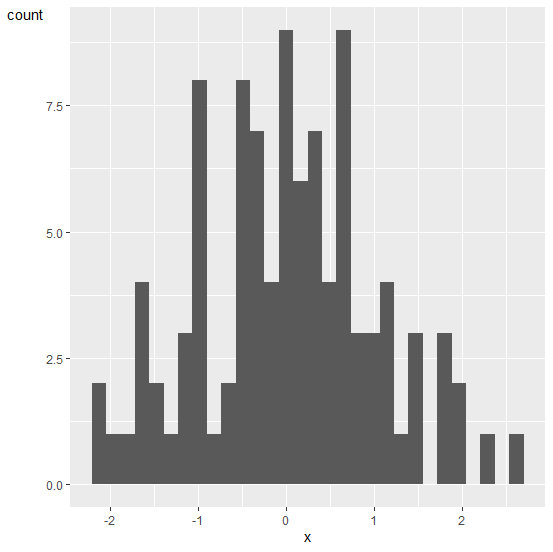# How to change the Y-axis title to horizontal using ggplot2 in R?

The default direction of Y-axis title using ggplot2 in R is vertical and we can change to horizontal. For this purpose, we can use theme function of ggplot2 package. We would need to use the argument of theme function as axis.title.y=element_text(angle=0)) and this will write the Y-axis title to horizontal but the position will be changed to top.

## Example

Live Demo

Consider the below data frame −

x<−rnorm(100)
df<−data.frame(x)
head(df,20)

## Output

x
1 −0.5417569
2 −0.4018531
3 0.2178570
4 1.1911282
5 0.5977775
6 0.5790850
7 −0.5143645
8 0.5056482
9 1.1791990
10 −0.2668907
11 −0.4142607
12 −0.3763989
13 0.6037290
14 −0.4162111
15 −0.1434954
16 1.9538955
17 0.2724839
18 1.7957378
19 1.9833760
20 −0.9305919

## Example

library(ggplot2)
ggplot(df,aes(x))+geom_histogram(bins=30)

## OutputChanging the Y−axis title direction to horizontal −

## Example

ggplot(df,aes(x))+geom_histogram(bins=30)+theme(axis.title.y=element_text(angle=0))

## Output